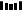### 题目描述

输入: [1,3,4,2,2]



输入: [3,1,3,4,2]



• 不能更改原数组（假设数组是只读的）。
• 只能使用额外的 O(1) 的空间。
• 时间复杂度小于 O(n^2) 。
• 数组中只有一个重复的数字，但它可能不止重复出现一次。

### 题目解析

[1,3,4,2,2]                      元素个数
<= 1 的元素：1                       1
<= 2 的元素：1, 2, 2                 3
<= 3 的元素：1, 2, 2, 3              4
<= 4 的元素：1, 2, 2, 3, 4           5


[3,1,3,4,2]
<= 1 的元素：1                        1
<= 2 的元素：1, 2                     2
<= 3 的元素：1, 2, 3, 3               4
<= 4 的元素：1, 2, 3, 3, 4            5


[3,3,3,3,4]
<= 1 的元素：                        0
<= 2 的元素：                        0
<= 3 的元素：3, 3, 3, 3              4
<= 4 的元素：3, 3, 3, 3, 4           5


• “如果选中的数 小于 我们要找的答案，那么整个数组中小于或等于该数的元素个数必然小于或等于该元素的值;

• 如果选中的数 大于或等于 我们要找的答案，那么整个数组中小于或等于该数的元素个数必然 大于 该元素的值”

### 代码实现一

//二分查找
class Solution {
public int findDuplicate(int[] nums) {
int len = nums.length;
int start = 1;
int end = len - 1;

while (start < end) {
int mid = start + (end - start) / 2;
int counter = 0;
for (int num:nums) {
if (num <= mid) {
counter++;
}
}
if (counter > mid) {
end = mid;
} else {
start = mid + 1;
}
}
return start;
}
}


### 代码实现二

//快慢指针
public int findDuplicate(int[] nums) {
int fast = nums[nums];
int slow = nums;

while (fast != slow) {
fast = nums[nums[fast]];
slow = nums[slow];
}

slow = 0;
while (fast != slow) {
fast = nums[fast];
slow = nums[slow];
}

return slow;
}1.程序员

2.【GitHub

3.【算法动画：七分钟理解什么是KMP算法

4.【数据结构十大经典排序算法动画与解析，看我就够了！▼ 点击『阅读原文』解锁更多图解 LeetCode 题目